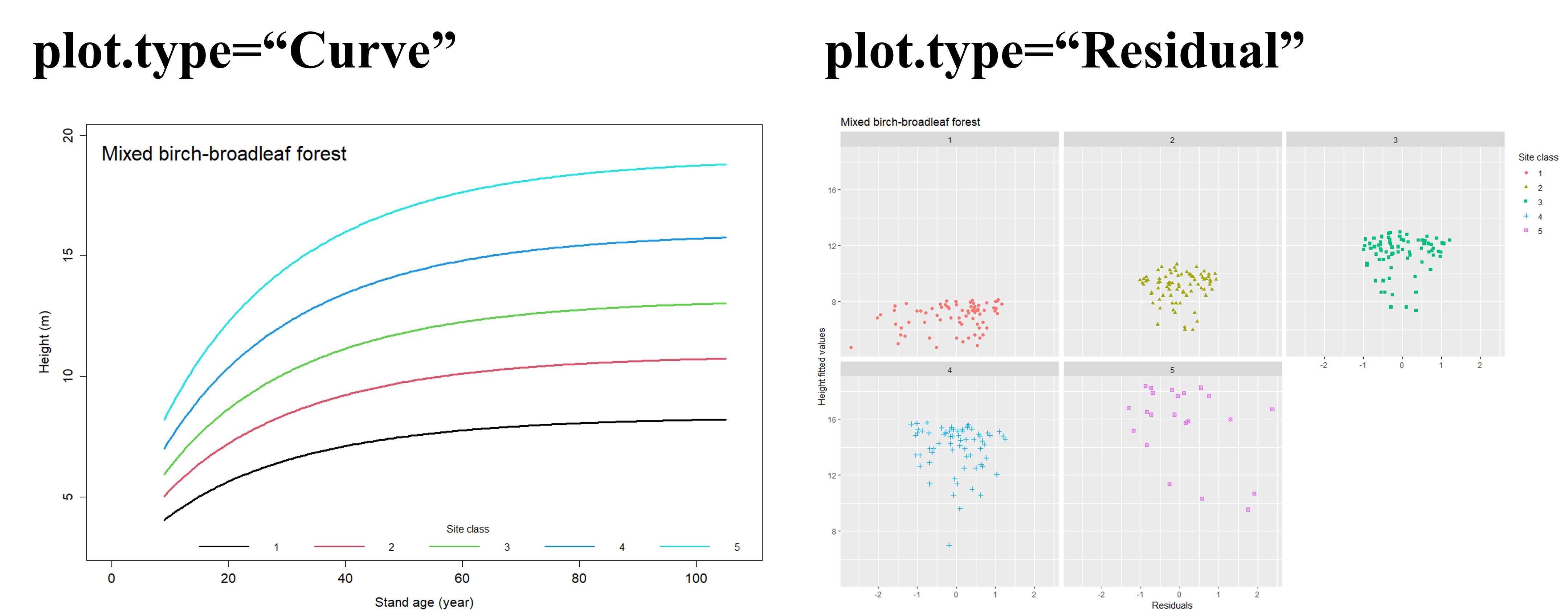# Forest Carbon Sequestration and Potential Productivity Calculation

Forestat version: 1.1.0

Date: 10/10/2023

`Forestat` is an R package based on `Methodology and Applications of Site Quality Assessment Based on Potential Mean Annual Increment`  and `A basal area increment-based approach of site productivity evaluation for multi-aged and mixed forests`  proposed by the Institute of Forest Resource Information Techniques, Chinese Academy of Forestry. This package can be used to classify site classes based on the stand height growth and establish a nonlinear mixed-effect biomass model under different site classes based on the whole stand model to achieve more accurate estimation of carbon sequestration. In particular, a carbon sequestration potential productivity calculation method based on the potential mean annual increment is proposed. This package is applicable to both natural forests and plantations. It can quantitatively assess stand’s potential productivity, realized productivity, and possible improvement under certain site, and can be used in many aspects such as site quality assessment, tree species suitability evaluation, and forest degradation evaluation.

## 1 Overview

`Forestat` can be used to implement the calculation of carbon sequestration potential productivity and the assessment of degraded forests. The calculation of carbon sequestration potential productivity includes the assessment of site classes based on stand height growth, establishment of the growth models of height (H-model), basal area at breast-height (BA-model), and biomass (Bio-model), as well as calculation of stand’s realized site productivity and potential productivity. The H-model can be constructed using Richard, Logistic, Korf, Gompertz, Weibull, and Schumacher model, while the BA-model and Bio-model can only be constructed using Richard model. The calculation of carbon sequestration potential productivity relies on data from several plots for a given forest type (tree species). The assessment of degraded forests relies on data from several trees and sample plots. Some sample datas are provided in the `Forestat` package.

### 1.1 forestat Flowchart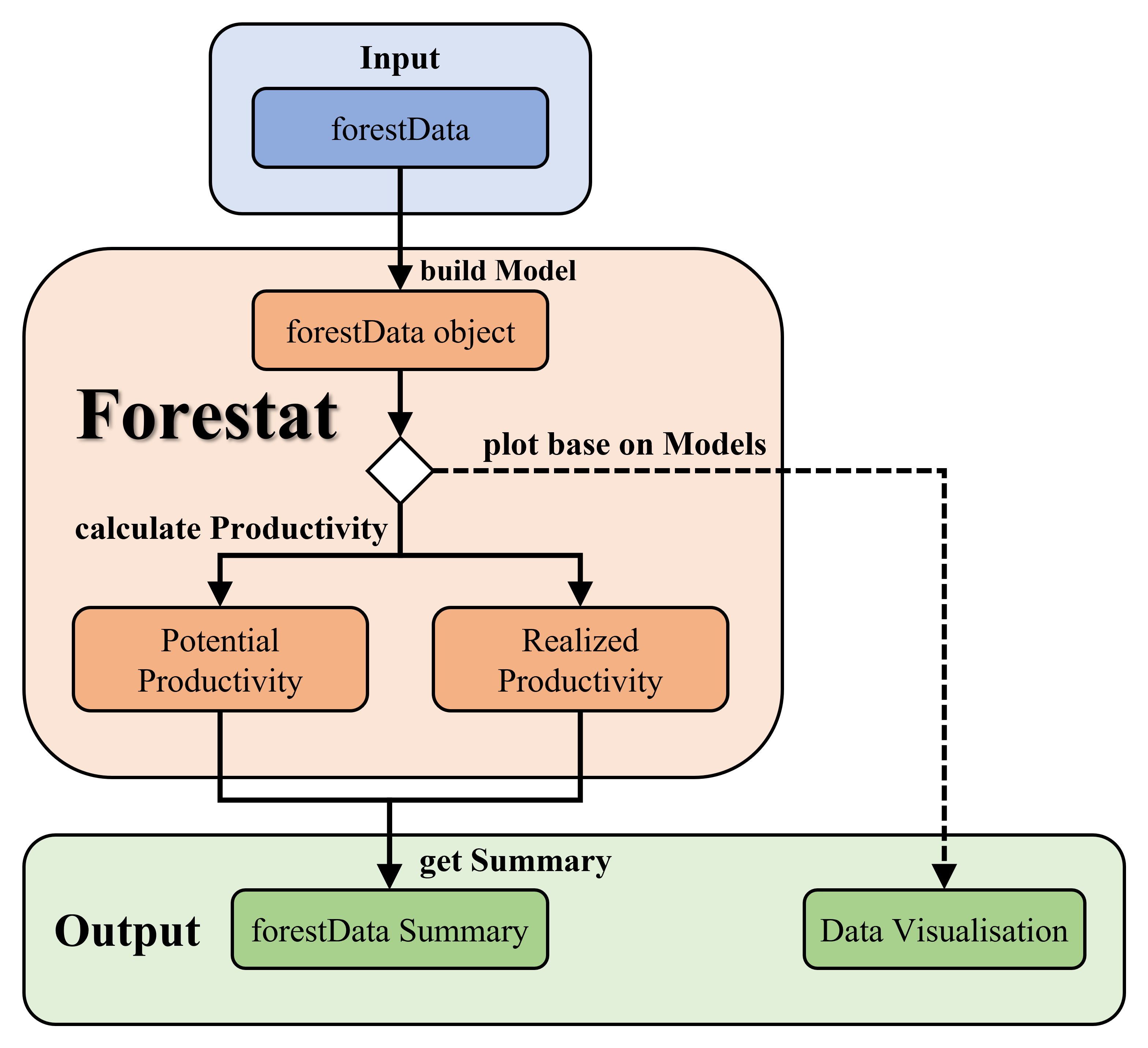Figure 1.1 Flowchart of the carbon sequestration potential productivity calculation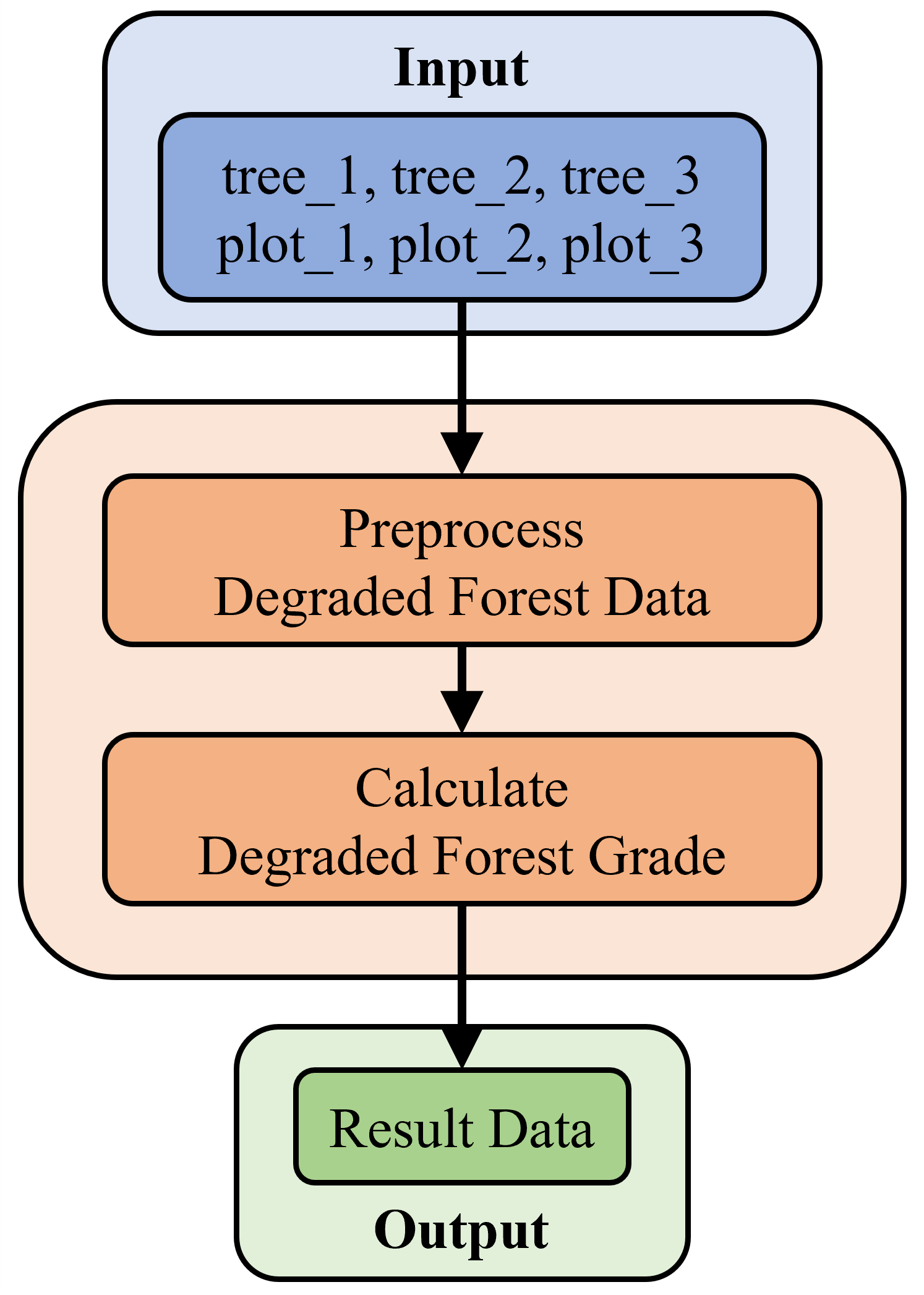Figure 1.2 Flowchart of degraded forest assessment

### 1.2 R Packages Required by forestat

dplyr https://CRAN.R-project.org/package=dplyr
ggplot2 https://CRAN.R-project.org/package=ggplot2
nlme https://CRAN.R-project.org/package=nlme

## 2 Installation

### 2.1 Install from CRAN or GitHub

To install `forestat` from CRAN in R, use the following command:

``````# Install forestat
install.packages("forestat")``````

Alternatively, you can install `forestat` from GitHub in R using the following command:

``````# Install devtools
install.packages("devtools")

# Install forestat
devtools::install_github("caf-ifrit/forestat/forestat")``````

``library(forestat)``

## 3 Quick Start

This part demonstrates the complete steps to perform the calculation of stand’s site classes, realized site productivity and potential productivity quickly using the sample dataset called `forestData` included in the package.

``````# Load the forestData sample data included in the package
data("forestData")

# Build a model based on the forestData and return a forestData class object
forestData <- class.plot(forestData, model = "Richards",
interval = 5, number = 5, H_start=c(a=20,b=0.05,c=1.0))

# Plot the scatter plot of the H-model
plot(forestData,model.type="H",plot.type="Scatter",
title="The H-model scatter plot of the mixed birch-broadleaf forest")

# Calculate the potential productivity of the forestData object
forestData <- potential.productivity(forestData)

# Calculate the realized productivity of the forestData object
forestData <- realized.productivity(forestData)

# Get the summary data of the forestData object
summary(forestData)``````

This part demonstrates the complete steps to perform the assessment of degraded forests using the sample data: tree_1, tree_2, tree_3, plot_1, plot_2, and plot_3 included in the package.

``````# Load the sample data tree_1, tree_2, tree_3, plot_1, plot_2, and plot_3 included in the package
data(tree_1)
data(tree_2)
data(tree_3)
data(plot_1)
data(plot_2)
data(plot_3)

# Preprocessing the degraded forest data
plot_1, plot_2, plot_3)

# View calculation results
res_data``````

## 4 Carbon Sequestration Potential Productivity Calculation

4.1 Build Model
4.1.1 Custom Data

To build an accurate model, high quality data is essential. The `forestat` package includes a cleaned sample dataset that can be loaded and viewed using the following command:

``````# Load the forestData sample data included in the package
data("forestData")

# Select the ID, code, AGE, H, S, BA, and Bio fields from the forestData sample data
# and view the first 6 rows of data
head(dplyr::select(forestData, ID, code, AGE, H, S, BA, Bio))

# Output
ID code AGE   H         S       BA       Bio
1  1    1  13 2.0 152.67461 4.899382 32.671551
2  2    1  15 3.5  68.23825 1.387268  5.698105
3  3    1  20 4.2 128.32683 3.388492 22.631467
4  4    1  19 4.2 204.93928 4.375324 18.913886
5  5    1  13 4.2  95.69713 1.904063  6.511951
6  6    1  25 4.7 153.69393 4.129810 28.024739``````

Of course, you can also choose to load custom data:

``````# Load custom data

The data from customers is required to have the csv or excel xlsx format. The following columns or fields including ID (plot ID), code (forest type code of plot), AGE (the average age of stand), and H (the average height of stand) are required to build the H-Model and make the relevant example graphs.

The `S` (stand density index), `BA` (stand basal area), and `Bio` (stand biomass) are optional fields to build the `BA-model` and `Bio-model`.

In the subsequent calculation of potential productivity and realized productivity, the `BA-model` and `Bio-model` are required. That is, if the customized data lacks the `S`, `BA`, and `Bio` fields, potential productivity and realized productivity cannot be calculated.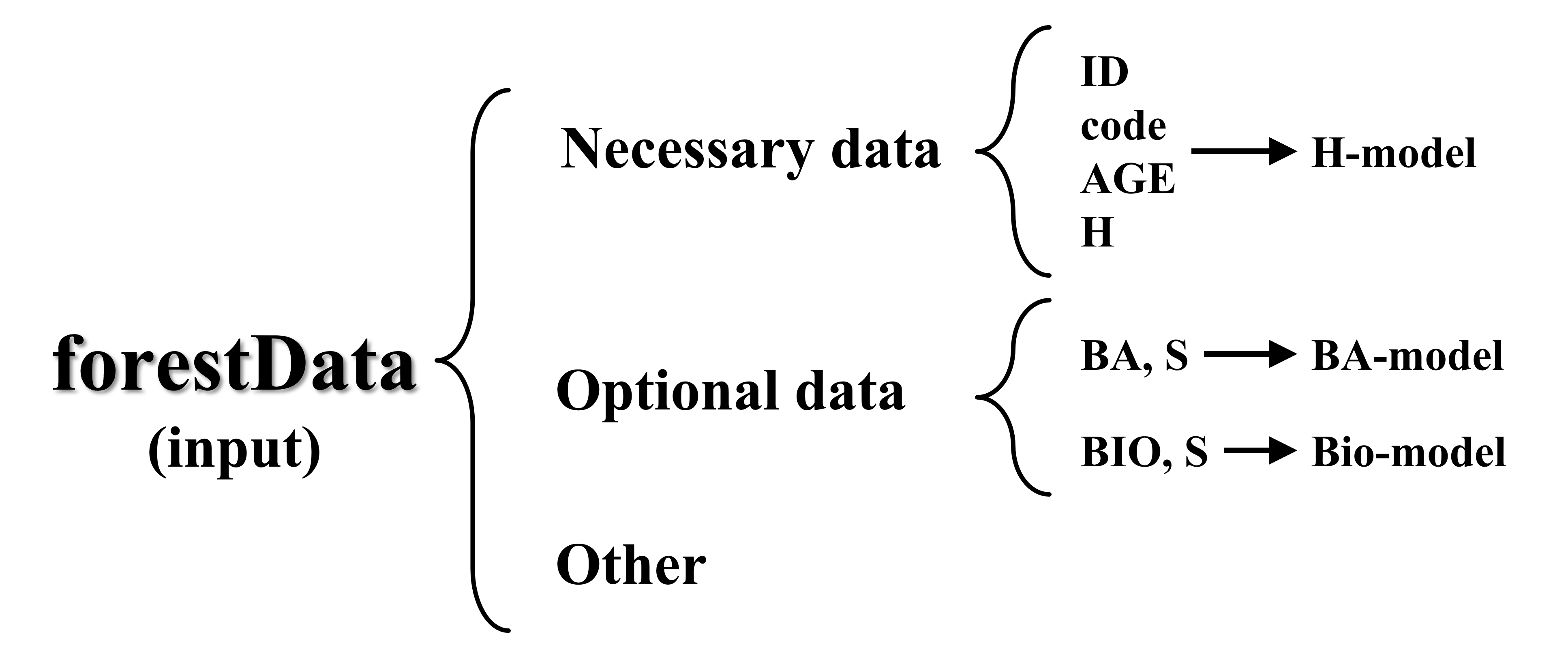Figure 2. Custom data format requirements

4.1.2 Build Stand Growth Model

After the data is loaded, `forestat` will use the `class.plot()` function to build a stand growth model. If the custom data contains the `ID, code, AGE, H, S, BA, Bio` fields, the `H-model`, `BA-model`, and `Bio-model` will be built simultaneously. If only the `ID, code, AGE, H` fields are included, only the `H-model` will be built.

``````# Use the Richards model to build a stand growth model
# interval = 5 indicates that the initial stand age interval for height classes is set to 5, number = 5 indicates that the maximum number of initial height classes is 5, and maxiter=1000 sets the maximum number of model fitting iterations to 1000
# The initial parameters for H-model fitting is set to H_start=c(a=20,b=0.05,c=1.0) by default
# The initial parameters for H-model fitting is set to BA_start=c(a=80, b=0.0001, c=8, d=0.1) by default
# The initial parameters for H-model fitting is set to Bio_start=c(a=450, b=0.0001, c=12, d=0.1) by default
forestData <- class.plot(forestData, model = "Richards",
interval = 5, number = 5, maxiter=1000,
H_start=c(a=20,b=0.05,c=1.0),
BA_start = c(a=80, b=0.0001, c=8, d=0.1),
Bio_start=c(a=450, b=0.0001, c=12, d=0.1))``````

The `model` parameter is the model used to build the `H-model`. Optional models include `"Logistic"`, `"Richards"`, `"Korf"`, `"Gompertz"`, `"Weibull"`, and `"Schumacher"`. The `BA-model` and `Bio-model` are built using the Richard model by default. `interval` parameter is the initial stand age interval for height classes, `number` parameter is the maximum number of initial height classes, and `maxiter` parameter is the maximum number of fitting iterations. The `H_start` is the initial parameter for fitting the H-model, the `BA_start` is the initial parameter for fitting the BA-model, and the `Bio_start` is the initial parameter for fitting the Bio-model. If fitting encounters errors, you can try different initial parameters as attempts.

The result returned by the `class.plot()` function is the `forestData` object, which includes `Input` (input data and height classes results), `Hmodel` (H-model results), `BAmodel` (BA-model results), `Biomodel` (Bio-model results), and `output` (Expressions, parameters, and precision for all models).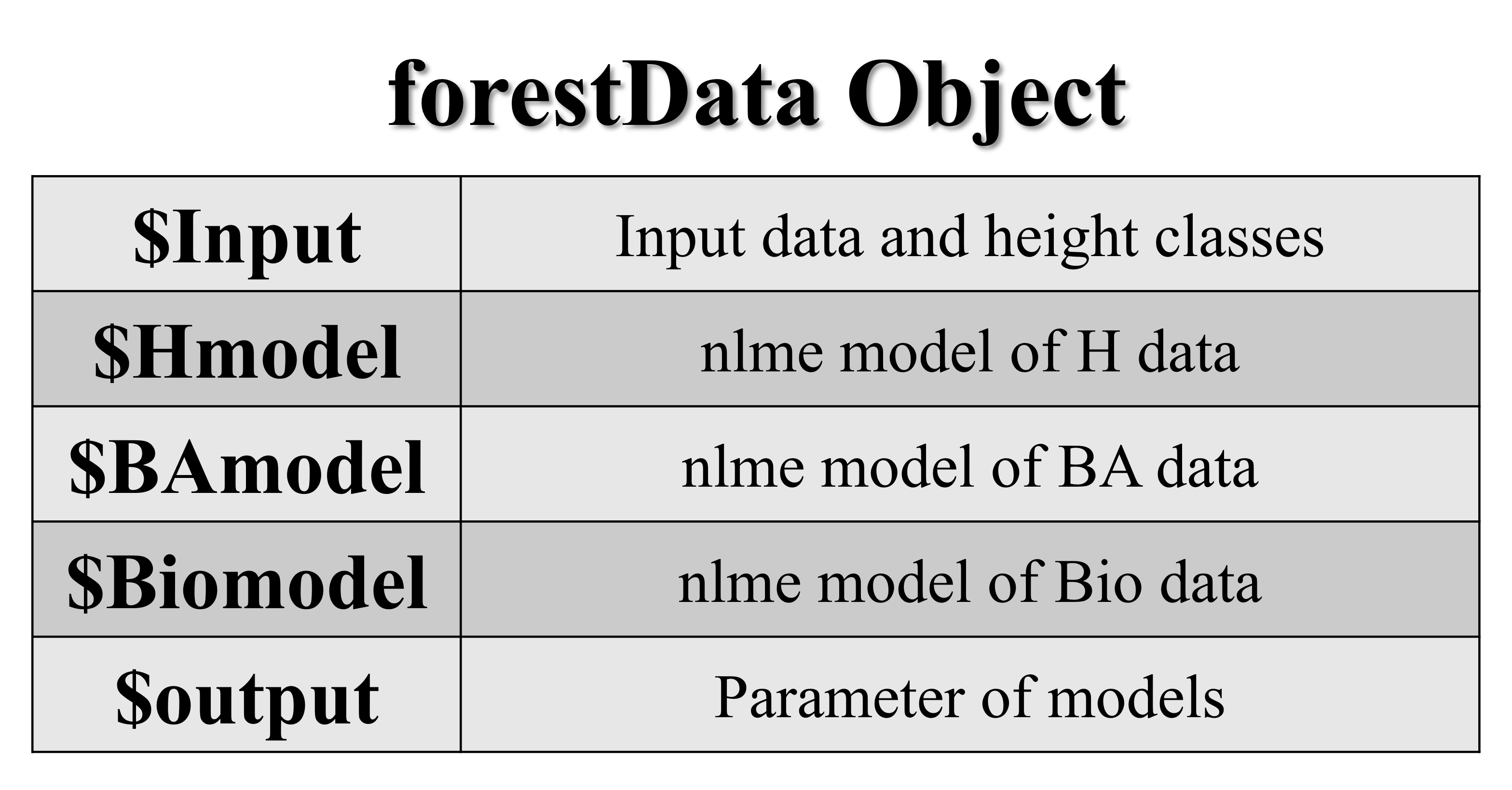Figure 3. Structure of the forestData object

4.1.3 Obtain Summary Data

To understand the establishment of the model, you can use the `summary(forestData)` function to obtain the summary data of the `forestData` object. The function returns the `summary.forestData` object and outputs the relevant data to the screen.

The first paragraph of the output is the summary of the input data, and the second, third, and fourth paragraphs are the parameters and concise reports of the `H-model`, `BA-model`, and `Bio-model`, respectively.

``summary(forestData)``
``````# Output
# First paragraph
H               S                 BA              Bio
Min.   : 2.00   Min.   :  68.24   Min.   : 1.387   Min.   :  5.698
1st Qu.: 8.10   1st Qu.: 366.37   1st Qu.: 9.641   1st Qu.: 52.326
Median :10.30   Median : 494.76   Median :13.667   Median : 78.502
Mean   :10.62   Mean   : 522.53   Mean   :14.516   Mean   : 90.229
3rd Qu.:12.90   3rd Qu.: 661.84   3rd Qu.:18.750   3rd Qu.:115.636
Max.   :19.10   Max.   :1540.13   Max.   :45.749   Max.   :344.412

# Second paragraph
H-model Parameters:
Nonlinear mixed-effects model fit by maximum likelihood
Model: H ~ 1.3 + a * (1 - exp(-b * AGE))^c
Data: data
AIC      BIC    logLik
728.4366 747.2782 -359.2183

Random effects:
Formula: a ~ 1 | LASTGROUP
a  Residual
StdDev: 3.767163 0.7035752

Fixed effects:  a + b + c ~ 1
Value Std.Error  DF  t-value p-value
a 12.185054 1.7050081 313 7.146625       0
b  0.037840 0.0043682 313 8.662536       0
c  0.761367 0.0769441 313 9.895060       0
Correlation:
a      b
b -0.110
c -0.093  0.946

Standardized Within-Group Residuals:
Min           Q1          Med           Q3          Max
-3.858592084 -0.719253472  0.007120413  0.761123585  3.375793806

Number of Observations: 320
Number of Groups: 5

Concise Parameter Report:
Model Coefficients:
a1       a2       a3       a4       a5          b         c
7.013778 9.575677 11.90324 14.67456 17.75801 0.03783956 0.7613666

Model Evaluations:
pe      RMSE        R2       Var       TRE      AIC      BIC    logLik
-0.006484677 0.6980625 0.9543312 0.4887767 0.3960163 728.4366 747.2782 -359.2183

Model Formulas:
Func                  Spe
model1:H ~ 1.3 + a * (1 - exp(-b * AGE))^c model1:pdDiag(a ~ 1)

# Third paragraph (similar data format to the second paragraph)
BA-model Parameters:

# Omitted here
......

# Fourth paragraph (similar data format to the second paragraph)
Bio-model Parameters:

# Omitted here
......``````

4.2 Make Graphs

After constructing the stand growth model using the `class.plot()` function in 4.1.2, you can use the `plot()` function to make graphs.

The `model.type` parameter specifies the model used for plotting, which include `H`, `BA`, or `Bio`. The `plot.type` parameter specifies the type of plot, which can be `Curve`, `Residual`, `Scatter_Curve`, or `Scatter`. The `xlab`, `ylab`, `legend.lab`, and `title` parameters represent the x-axis label, y-axis label, legend, and title of the graph, respectively.

``````# Plot the curve of the H-model
plot(forestData,model.type="H",
plot.type="Curve",
xlab="Stand age (year)",ylab="Height (m)",legend.lab="Site class",
title="The H-model curve of the mixed birch-broadleaf forest")

# Plot the scatter plot of the BA-model
plot(forestData,model.type="BA",
plot.type="Scatter",
xlab="Stand age (year)",ylab=expression(paste("Basal area ( ",m^2,"/",hm^2,")")),legend.lab="Site class",
title="The BA-model scatter plot of the mixed birch-broadleaf forest")``````

The sample plots produced by different `plot.type` values are shown in Figure 4: